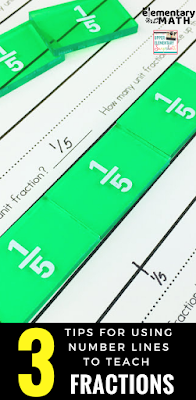# 3 Tips for Using Number Lines to Teach FractionsUnderstanding fractions can be quite difficult for some of our kids.

Number lines have always been effective tools to teach different operations like addition, subtraction and multiplication. These trusty lines also come in handy when understanding how to count, compose and compare fractions (just to name a few).

Much like a ruler, a number line is a linear scale that’s divided into equal parts.  They are great tools because both whole numbers and fractions live on them. However, students often don't think of fractions as numbers, making it challenging for them to understand.

To support our students in understanding number lines and fractions, I’ve come up with 3 tips.

Tip #1: Focus on the Distance NOT the Hash Marks

In my experience, a lot of kids count the hash marks on a number line starting with 0, leading to major misconceptions.

The example below shows how a student may incorrectly label points due to counting hash marks.

Starting with 0, they count all the hash marks on the number line. In this case 5. Then they use that number to represent the denominator.

From there, they "find" the numerator by counting the hash marks up until the designated point. In this case they count 3, which becomes the numerator.  Finally, students determine the designated point is 3/5 and not 2/4.

Based on the example above you can see how counting hash marks (without understanding what they represent) causes errors.  It’s very important that we show kids how to correctly read number lines represented by fractions.

In our everyday lives, we use tools like rulers and tape measures to determine the length of objects.  Much like these tools, number lines should be used and read in the same way.  Students should start from 0 and look at the distance to a designated point.

For example, when trying to find the point 2/4 on the number line students should:

FIRST - count the number of equally divided parts between 0 and 1 on the number line (i.e. 4 equal segments

NEXT - determine what fraction is represented by one equal segment (i.e. ¼)

THEN - determine the distance from 0 to the designated point by counting and combining the unit fractions. (i.e. two one fourth parts or ¼ + ¼ = 2/4)

Determining the distance from 0 to a designated point is an important discovery for kids and may take time and practice to develop.

Tip #2: Use Fraction Bars or Tiles along with Number Lines

Fraction bars or tiles are great visual aids that allow kids to see fractions as a part of a whole number. They also serve as great hands on tools.

Teacher Tip:  I’ve found that before giving any assignment that involves manipulatives, it’s important to give students time to freely explore them. Three - five minutes of free exploration is worth the time you get back.

As a quick task, ask students to build a fraction using fraction bars. Guiding questions or prompts can include:

•         Make 3/5 using the fraction bars
•         How do we know that your model represents 3/5?
•         What unit fraction did you use to build that fraction?
•      How many unit fractions were used to build that fraction?
•         Let’s draw a number line under the fraction bars and partition it.
•        How is the number line similar to the fraction representation? How is it different?

Give your students plenty of opportunities to compose fractions in this way. You can also vary the task, by giving students opportunities to build other fractions that are equal to 1 and beyond 1.

These tasks are sure to leave a lasting impression because of the hands-on nature.

Tip #3: Engage Kids in Fun Games and Activities

Now that your students understand how to use the number line to identify fractions, give them a chance to apply the skill in a fun way.

Using games to reinforce fractions on a number line helps stimulate their minds and keep them engaged.  For this reason, I've created Fractions on a Number Line Games and Activities.  This pack has 3 fun fraction games plus a variety of activity sheets.

One of these games is called Face Off  and kids LOVE it, because they want to be the first player to correctly match their game card with the number line on the game board.

As we know, students learn by doing and what better way to make this happen than through games?

These games and practice sheets are great for math centers or as an early finisher partner activity.

Make teaching fractions fun and engaging by using number lines. Use tools like math games and fraction bars to involve your students in the learning process.  I hope that you use some of these tips during your next math lesson.

Want to find the fraction games mentioned earlier? Be sure to check them out here.